# Image Compositing in Computer Graphics

Written by Paul Bourke
December 2002

Compositing is the name given to the process of combining multiple images as overlapping layers producing a single output image. An early use of compositing was in the cartoon industry where each artist draws one aspect of the scene as a layer, the multiple layers from each artist are combined to form the current frame. Traditionally the layers were drawn on transparent sheets which could simply be overlaid and photographed. What the advent on computer based cartoon animation the regions of the layers with content were identified with a so called alpha channel, the alpha channel might be 0 for those regions which are not drawn upon to indicate they are transparent. The alpha channel might be 1 for the regions that are drawn upon to indicate they are opaque. This gave rise to the rgba colour space, that is, each pixel has 4 bytes one for each of red, green, blue, and alpha. For example, a rgba image is shown below, on the left is the RGB image (it only has blue and white pixels) and on the right is one possible corresponding alpha channel.

 RGB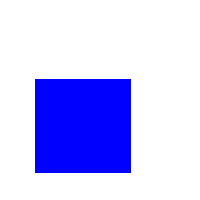Alpha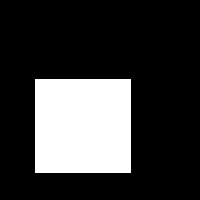Image 1

With an alpha channel indicating opaque (1, white) or transparent (0, black), layers can now be added together from the background to the foreground such that those parts of a layer that are transparent don't affect anything behind them and those parts that are opaque write over anything behind them. For example, when combining a pixel in the foreground layer P2 = (R2,G2,B2,A2) with the corresponding pixel in the background layer P1 the following expression will achieve the desired effect. Where the foreground image has an alpha value of 1 the final contribution comes entirely from the foreground image. Where the foreground image has an alpha value of 0 the final contribution is entirely from the background image.

 R = R1 (1 - A2) + R2 A2 G = G1 (1 - A2) + G2 A2 B = B1 (1 - A2) + B2 A2 A = 1

An example of this is shown below, consider the image above of the blue rectangle as the background image and composite onto that the following layer.

 RGB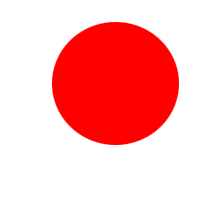AlphaImage 2

The result according to the above equations will be as follows.

 RGB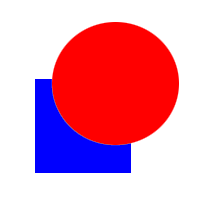AlphaImage 3

The discussion above has been limited to completely opaque or totally transparent layers. While that is all that is necessary in many cases, there are many other applications that require various levels of transparency. This arose in computer generated cartoon animation where antialiasing is required, this requires a range of alpha values on the antialiased edges. As another example, one might want to create the effect of semi-transparent coloured glass where the colour of the foreground pixels modifies the colour of the background layers. For example the red circle in the above example, if it were 50% transparent might give the following image when composited onto the blue square in image 1.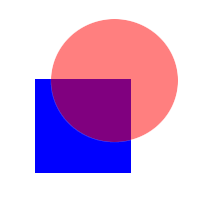Image 4

As soon as there is a more continuous value for the alpha channel, using all the possible 256 levels that can be stored in 1 byte, then there are many more ways of combining a pair of images. These are typically called blending functions and there are quite a few in common usage depending on the desired effect. For a description of a very powerful set of blending functions see the OpenGL API documentation. For example all the following 3 images are derived by combining image 1 and image 2 with different functions. Note that this is by no means an exhaustive set, many others are possible and in common usage.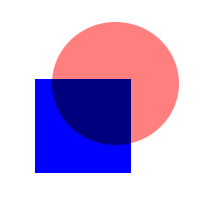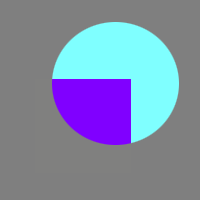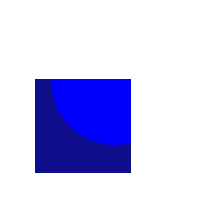Image 5

Perhaps the most common blending function was first promoted by Alvy Smith and Ed Catnull around 1977 and is often called the "over" operator.

 R = R1 (1 - A2) + R2 A2 G = G1 (1 - A2) + G2 A2 B = B1 (1 - A2) + B2 A2 A = A1 (1 - A2) + A2 A2

The image on the right is an example of using this blending function to composite 4 layers, each has a different colour square and the regions within each square have a different but constant alpha value.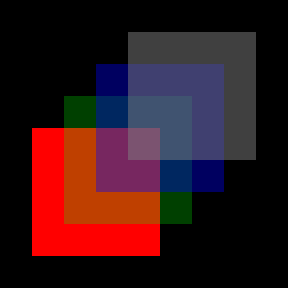For some application there is a serious problem with the above blending function, it is not associative. That is, if we have 4 layers to composite the result from compositing (A & B & C & D) is not the same as (A & B) & (C & D), indeed this is true for the vast majority of the blending functions. Having a blending function that is associative is often required (eg: distributed volume rendering) and in other cases can lead to improved compositing performance.

Bruce Wallace and Marc Levoy, and later, Tim Porter and Tom Duff proposed the following blending function that is associative. Note that the following expression can be simplified (and compositing performance increased) by premultiplying each colour by its alpha value.

 R = R1 A1 (1 - A2) + R2 A2 G = G1 A1 (1 - A2) + G2 A2 B = B1 A1 (1 - A2) + B2 A2 A = A1 (1 - A2) + A2